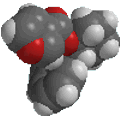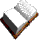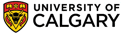Chapter 13: SpectroscopyMolecular ion

• The molecular ion is the ion produced when the molecule is ionised.
• The m/z of this ion corresponds to the molecular weight of the sample.
• The molecular ion should be the heaviest ion in the mass spectra (remembering to allow for the presence of isotopes such as 13C)
• Note that not all samples give a molecular ion.... the ion needs to be stable enough to survive until detection.
 IMPORTANT When calculating molecular weights to match to a molecular ion you need to use integer atomic weights. This is because relative atomic weights are based on the natural abundance of isotopes and since MS separates the isotopes, the peaks appear at different m/z values.

The nitrogen rule

Organic molecules composed of C, H, O and halogens have even molecular weights
Examples : CH4 = 16 g/mol, CH3CH3 = 30 g/mol, CH3OH = 32 g/mol, CH3F = 34 g/mol, CH3CO2H = 60 g/mol

Since N forms 3 bonds, compounds with an odd number of N atoms, have odd molecular weights
Examples : NH3 = 17 g/mol, CH3NH2 = 31 g/mol, C5H5N = 79 g/mol

Therefore, the implication is the nitrogen rule.... "an odd molecular weight implies the presence of an odd number of N atoms" .
This can be a useful piece of information and prompt you to look for N containing functional groups in the IR spectrum.© Dr. Ian Hunt, Department of Chemistry